Courses
Courses for Kids
Free study material
Free LIVE classes
More

# How Many Squares Class 5 Notes CBSE Maths Chapter 3 (Free PDF Download)LIVE
Join Vedantu’s FREE Mastercalss

## How Many Squares - NCERT Maths Class 5 Chapter 3 Revision Notes and Worksheets

You have previously learned about squares and their properties. In Class 5 Maths Chapter 3, you will learn the advanced concepts of squares and how to determine areas with them. In this chapter, you will be taught how to draw squares and how to determine their number in a diagram. Download and refer to the How Many Squares revision notes prepared by the highly experienced teachers of Vedantu.

These notes comprise a simple explanation of the concepts related to squares and defining an area with them. You will also use the concepts of the perimeter of squares and rectangles given in the questions with figures. You can refer to online and download How Many Squares Class 5 PDF revision notes for free from Vedantu.

Last updated date: 23rd Sep 2023
Total views: 143.7k
Views today: 3.43k

## 2 Dimensional Figures / 2D Figures

• A two-dimensional shape is a plane figure that can be drawn on a flat surface.

• It has only two dimensions which are length and width. It has no thickness or depth

• Example: Rectangle, Circle, Square etc

### Area:

• The area is the region enclosed by the boundary of a closed figure.

• Square units are used to measure area.

### Perimeter:

• Perimeter is the length that forms the boundary of an area (or) the total length of the boundary is called Perimeter.

• If the figure is a triangle, square, or rectangle, the perimeter is the sum of the edge lengths.

• We measure the perimeter of any figure in mm, cm, m, or km.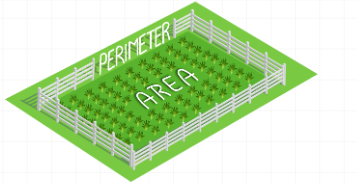Perimeter

### Dimensions:

• The measurement like length, width, and height of any object is known as dimensions. Different objects and shapes have different dimensions.

• Based on dimension the area and perimeter will differ which means even if they look similar their areas and perimeters will be different because of dimensions.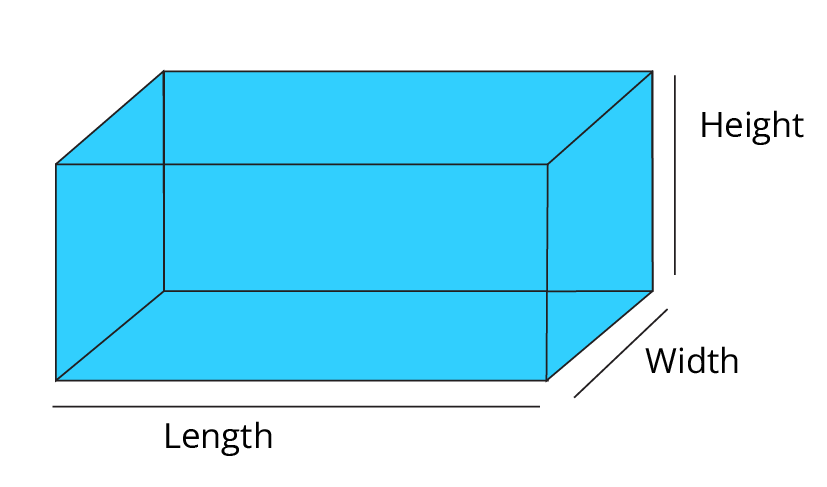Cuboid

Ans: Yes, on comparing my hand with my father’s palm his palm is bigger than mine and also my father’s hand is longer than my hand.Comparing Hands

### Counting Grids

• To find the area and perimeter of any object we use the counting grids method.

• The area of a plane figure is the number of squares required to completely cover it and the perimeter of a plane figure is the number of squares required to cover the boundary of the figure.

• Suppose we want to find the area of the below-given square. We can simply count the total number of small squares present in it because the area is nothing but space occupied by the shape of the object.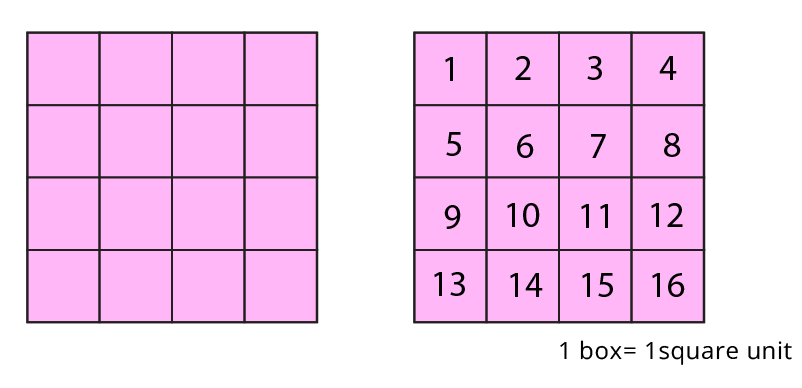Counting Grid

• Since it would be silly to always count all those squares to find the area and perimeter of any object. Also counting the squares will be difficult for the objects or shapes that big, that's why we use specific formulas for area and perimeter for specific objects which makes us easy to find.

### Square

• A closed, two-dimensional shape with 4 equal sides is known as a Square.

• In Square all 4 sides are equal

• Example of Square Shape Objects: Chessboard, a slice of bread, etc.

• Formula of area of a Square = side × side

• Formula of a Perimeter of a Square = 4 × length of a sideSquare

Solved Example 2: Find the perimeter and area of a square of side 10 cm.

Ans: We know that the perimeter of square = 4 × length of a side

Here given side= 10 cm

Therefore, perimeter = 4 × 10 cm = 40 cm

Now, area of the square = side × side sq. units

Therefore the area of square =10 × 10 = 121 cm2

### Rectangle:

• A Closed two-dimensional shape in geometry, with 4 sides and 4 corners is known as a Rectangle.

• In Rectangle opposite sides of a rectangle have the same lengths and are parallel.

• Example of Rectangle Shape Objects: Mobile,Television,Books etc.

• Formula of area of rectangle =length × breadth

• Formula of Perimeter of  Rectangle = 2 × length + 2 × breadth or 2(length + width).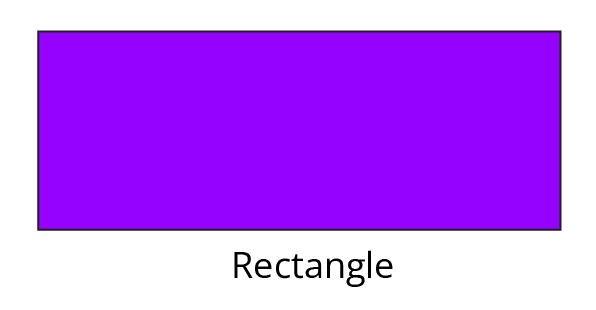Solved Example 3: Find the perimeter of a rectangle whose length and breadth are 10 cm and 12 cm, respectively.

Ans: Given: Length = 10 cm and Breadth = 12 cm

We know,

The perimeter of a rectangle = 2(length + width)

Substitute the value of length and width here,

Perimeter (P) = 2(10 + 12) cm

Thus p = 2 × 22 cm

Therefore, the perimeter of a rectangle = 44 cm.

Solved Example 4: Find the area of a rectangle with a length of 15 mm and a breadth of 9 mm.

Ans: Given

The Length of a rectangle = 15 mm.

The breadth of a rectangle = 9 mm.

We know that the area of a rectangle = length × breadth

Therefore the area of a rectangle = length × breadth

= 15 × 9 sq mm.

= 135 sq mm.

Thus the area of rectangle is 135 sq.mm

### Triangle:

• A triangle is a shape formed when three straight lines meet. All triangles have three sides and three corners (angles).

• Examples of triangles in real life: Pyramids, Sailing Boats, Sandwich and pizza slices etc

• Formula of area of triangle = $\frac{1}{2}$ × l × b where l = length and b = breadth.

• Formula of Perimeter of  triangle = a + b + c where a, b, c are sides.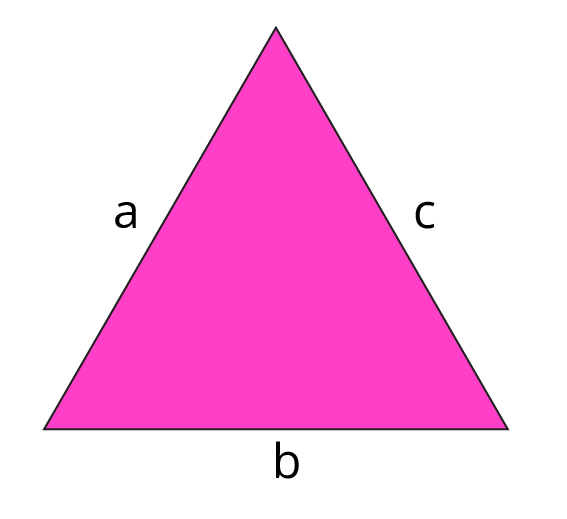Perimeter of Triangle

Solved Example 5: Find the area of a triangle with a base of 5 inches and a height of 8 inches.

Ans: Now let us find the area using the area of triangle formula:

We know that area of triangle = $\frac{1}{2}$ × l × b

Thus the area of triangle (A) = $\frac{1}{2}$ x 5 x 8

A = $\frac{1}{2}$ x 40

Therefore, the area of the triangle (A) =20 m2

Solved Example 6: Anshu wants to find the perimeter of the pyramid toy which is in a triangle shape each side is 12 cm.

Ans: Since all three sides are equal in length, the triangle is an equilateral triangle.

i.e. a = b = c = 12 cm

Perimeter = a + b + c

Perimeter = 12 + 12 + 12

Perimeter = 36

Perimeter = 36 cm.

## Relationship Between Area and Perimeter

• Let us consider two rectangles A and B having the same perimeter as 18 units.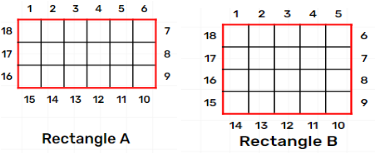Relationship Between Area and Perimeter of a Rectangle

Observe the given rectangles here both of them have different areas i.e, Area of Rectangle A = 18 square units and Area of Rectangle B is 20 square units.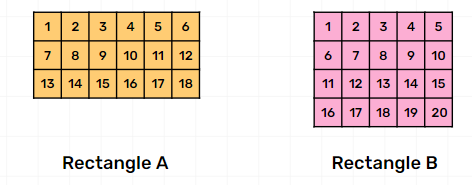Relationship Between Area and Perimeter

Hence We Conclude That:

• The two shapes having the same perimeter can have different areas.

• Similarly, two shapes having the same areas can have different perimeters.

## Area of Irregular Shapes

• Areas of irregular shapes can be found by counting the number of squares occupied by the irregular shape.

• As we don't have particular formulas for calculating the irregular shapes we use the counting square grids method.

• Below are the measures that we consider to find the area of irregular shapes.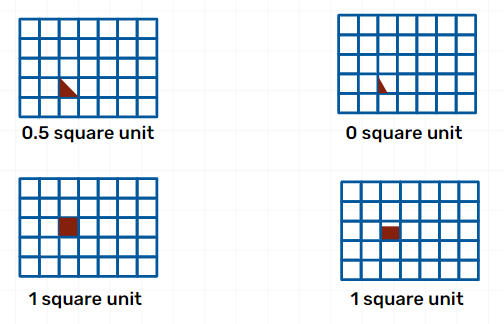Area of Irregular Shapes

Solved Example 7:  Find The Area of below Figure: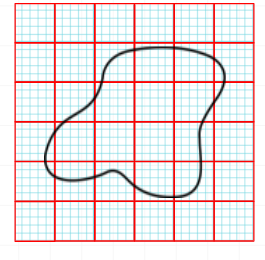Ans: After coloring and counting the squares we observe that the total area of the figure will be (3+7+1) square units = 11 square units.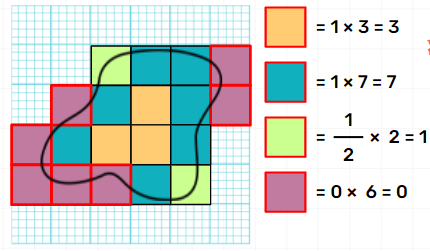### Patterns

• Patterns are a set of numbers arranged in a sequence such that they are related to each other in a specific rule.

• Patterns are repeated arrangements of shapes, colours and numbers which are arranged while rotating it.

• Example: tiles in our houses, checks on a shirt, mom’s saree with a design, a beautiful dress has a design pattern etc.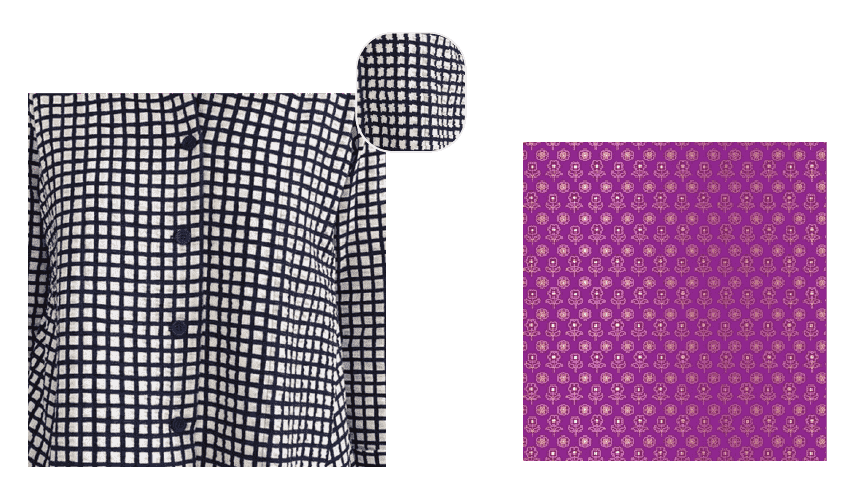## Practise Problems

1. A square field has a side, a = 2.5 m, What is its Area?

Ans: 6.25 m2

2. The length of a rectangular swimming pool is 10 metres and the breadth is 8 metres. Find the area of the swimming pool?

Ans: 2.80 m2

## Importance of CBSE Class 5 Maths Chapter 3 How Many Squares

As mentioned earlier, this chapter will focus on delivering knowledge related to squares, rectangles, and how to determine the area using squares. Here is the list of points that depict the importance of this chapter in the Class 5 Maths curriculum.

• It will describe how equal squares can fit into a big rectangle. By using this concept, you can determine the area of any rectangle. This fundamental concept will help you to understand how lengths and breadths of squares and rectangles are determined to deduce the areas.

• The How Many Squares summary teaches us to use a sheet with smaller equal squares on it to determine the area of irregular shapes such as handprints and footprints.

• It will also impart knowledge of how bigger rectangles are used rather than smaller squares to determine bigger areas easily.

## Benefits of How Many Squares Class 5 Worksheets and Revision Notes

• The subject experts at Vedantu have explained the fundamental concepts of this chapter in an easy-to-understand manner to help you understand them better.

• Clarify your doubts by referring to these explanations on your own and prepare for the exams.

• Revise the principles related to regular geometric shapes efficiently with the help of these revision notes.

• Solve the Class 5 Maths Chapter 3 How Many Squares worksheets to practice and sharpen your problem-solving skills in geometry.

• Develop a strong foundation of geometric concepts before you move to learn advanced theorems in higher classes.

## Prepare for the Exams with NCERT Class 5 Chapter 3 Maths Revision Notes

Download these free revision notes PDF and worksheets to prepare for your exams. You can also refer to the NCERT Solutions given by our subject experts and learn to figure out How Many Squares are there in the given figure. Get knowledge of how smaller square units are used to determine bigger areas.

Solve How Many Squares Class 5 worksheets PDF and verify your answers with the solutions provided. Learn the proven techniques to solve the questions given in this chapter to score well in the exams.

## Conclusion

"How Many Squares" chapter in CBSE Class 5 Maths is an essential and engaging topic that introduces students to the fascinating world of geometry and pattern recognition. Throughout the chapter, students explore the concept of squares and their various orientations, developing a deeper understanding of spatial reasoning and critical thinking. By identifying patterns and counting squares in different arrangements, students enhance their problem-solving skills and logical reasoning abilities. The free PDF download of Class 5 Notes on this topic provides valuable resources for students to reinforce their learning and practice independently. Mastering this chapter lays a solid foundation for their future mathematical journey and overall cognitive development.

## FAQs on How Many Squares Class 5 Notes CBSE Maths Chapter 3 (Free PDF Download)

1. Can I measure areas of irregular shapes?

NCERT Maths Class 5 Chapter 3 explains how the irregular shapes are plotted on paper with smaller equal squares drawn on them. Start counting the squares and add them to get the rough area of an irregular shape.

2. How to learn concepts related to areas of rectangles and triangles?

It is easier to learn how to determine the area of triangles and rectangles using smaller square units. You will also be able to correlate these fundamental concepts with advanced geometrical theorems later.